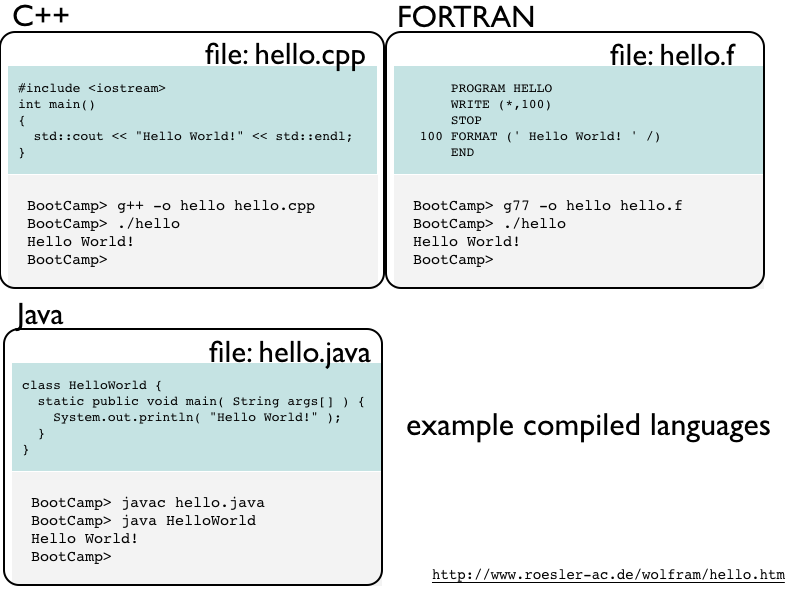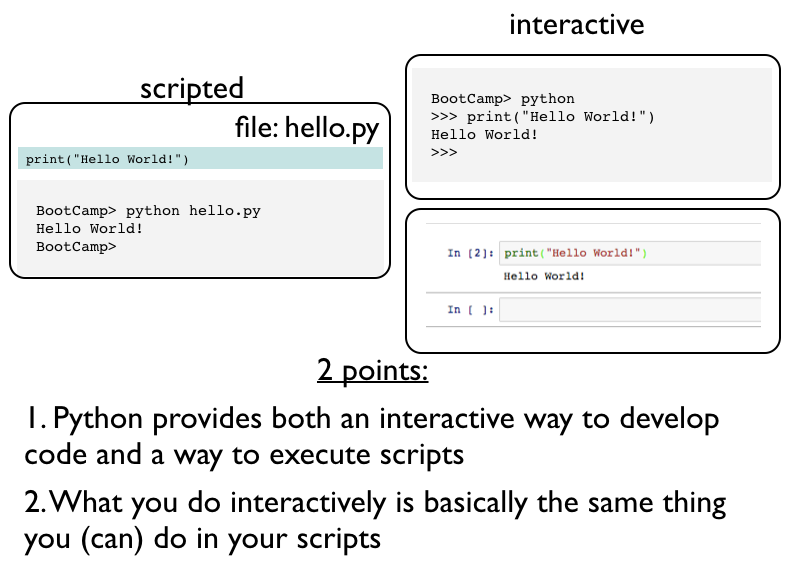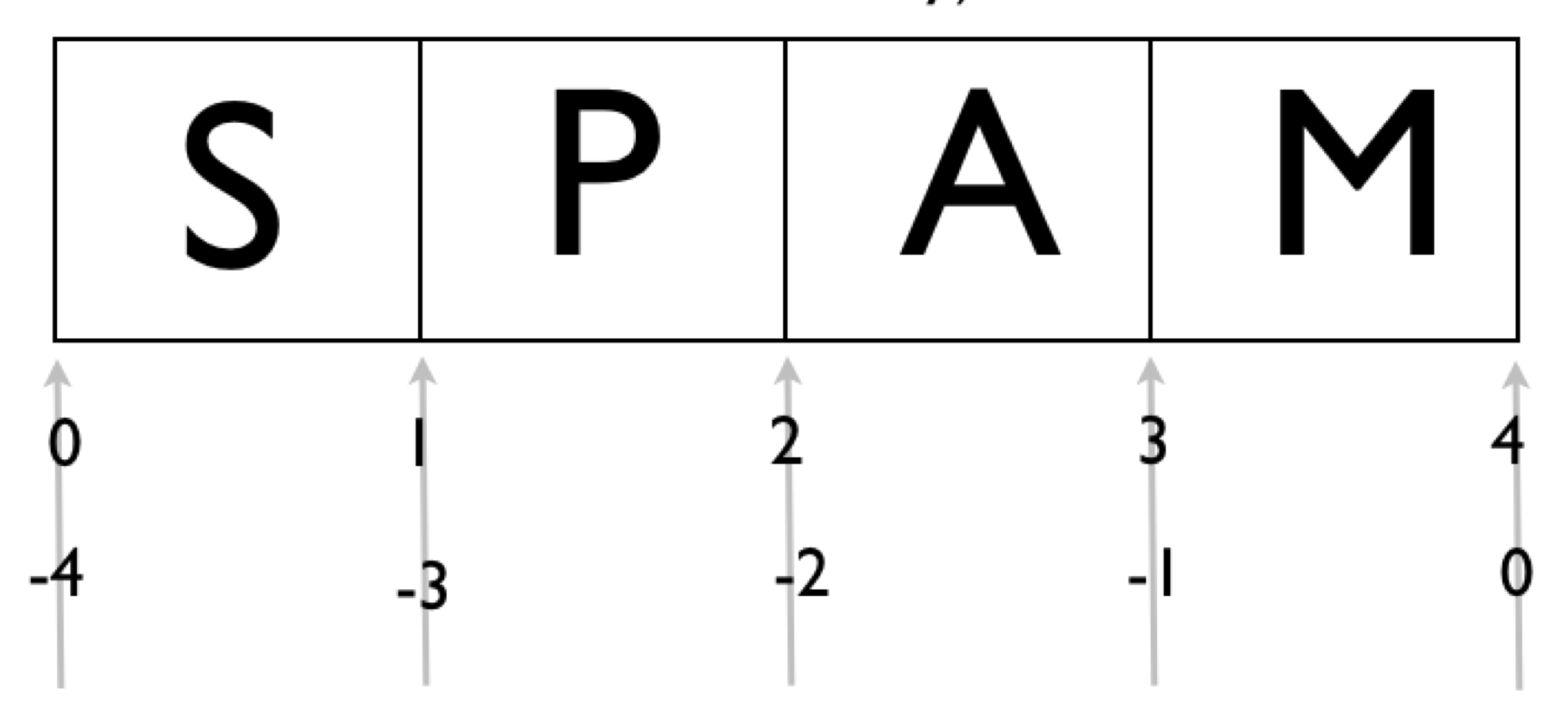## Basic Training¶

UC Berkeley Python Bootcamp


</pre>
follow along the code at:
http://bit.ly/pyboot_01


In :
%run talktools.py

In :
from IPython.display import YouTubeVideo
YouTubeVideo("imhrDrE4-mI",width="640",height="390")

Out:

### Outline¶

• Hello World!
• calculator/basic math
• strings
• variables
• basic control statements
• indentation!In [ ]:
print("Hello, world.")


# Calculator¶

there are int and float (but not doubles)

In [ ]:
print(2 + 2)

In [ ]:
2 + 2

In [ ]:
print(2.1 + 2)

In [ ]:
2.1 + 2 == 4.0999999999999996

In [ ]:
%run talktools

• Python stores floats as their byte representation so is limited by the same 16-bit precision issues as most other languages

• In doing calculations, unless you specify otherwise, Python will store the results in the smallest-byte representation

1. Indentation matters!
2. When you mess up, Python is gentle
3. # starts a comments (until the end of the line)
In [ ]:
print(2 + 2)
2 + 2

In [ ]:
2 # this is a comment and is not printed

In [ ]:
# this is also a comment


Calculator

• all the math operators you'd expect, including ** for power.
• In Python 3, there is no distinction between int and long
In [ ]:
42**42

In [ ]:
(42**42).bit_length()

In [ ]:
bin(42**42)


Division always leads to a float

In [ ]:
2 / 2

In [ ]:
2 / 2.0


Note: This is an important difference between Python 2 and Python 3. Old-style division between ints can be done with a double slash //

In [ ]:
2 // 2

In [ ]:
3 // 2

In [ ]:
2.5 // 2 # egad, dont do this.


There is also complex types

In [ ]:
complex(1,2)

In [ ]:
1+2j

In [ ]:
1 + 2j - 2j


Note: Access to decimal (decimal fixed point and floating point arithmetic) and fraction types/operations is through built-in modules.

## Let's do some math¶

In [ ]:
(3.0*10.0 - 25.0)/5.0

In [ ]:
print(3.085e18*1e6)  # this is a Megaparsec in units of cm!

In [ ]:
t = 1.0      # declare a variable t (time)
accel = 9.8  # acceleration in units of m/s^2

In [ ]:
# distance travelled in time t seconds is 1/2 a*t**2
dist = 0.5*accel*t*t
print(dist) # this is the distance in meters

In [ ]:
dist1 = accel*(t**2)/2
print(dist1)

In [ ]:
dist2 = 0.5*accel*pow(t,2)
print(dist2)

• variables are assigned on the fly
• multiplication, division, exponents as you expect
In [ ]:
print(6 / 5) ; print(9 / 5)

In [ ]:
print(6 // 5) ; print(9 // 5) # remember double-slash integer division returns the floor

In [ ]:
6 % 5  # mod operator, get the remainder. x = (x // y)*y + x % y

In [ ]:
1 << 2 ## shift: move the number 1 by two bits to the left
##        that is make a new number 100 (base 2)

In [ ]:
5 >> 1 ## shift: move the number 5 = 101 (base 2) one to
## to the right (10 = 2)

In [ ]:
x = 2 ; y = 3  ## assign two variables on the same line!
x | y          ## bitwise OR

In [ ]:
x ^ y          ## exclusive OR (10 ^ 11 = 01)

In [ ]:
x & y          ## bitwise AND

In [ ]:
x = x ^ y ; print(x)

In [ ]:
x += 3 ; print(x)

In [ ]:
x /= 2.0 ; print(x)


we'll see a lot more mathy operators and functions later

## Relationships¶

In [ ]:
# from before dist1  = 4.9 and dist = 4.9
dist1 == dist

In [ ]:
dist < 10

In [ ]:
dist <= 4.9

In [ ]:
dist < (10 + 2j)

In [ ]:
dist < -2.0

In [ ]:
dist != 3.1415


Try using some Greek characters as variables:

\Delta

More on Variables & Types

In [ ]:
0 == False

In [ ]:
not False

In [ ]:
0.0 == False

In [ ]:
not (10.0 - 10.0)

In [ ]:
not -1

In [ ]:
not 3.1415

In [ ]:
x = None    # None is something special. Not true or false
None == False

In [ ]:
None == True

In [ ]:
False or True

In [ ]:
False and True

In [ ]:
float("nan") == True

In [ ]:
print(float('inf'))

In [ ]:
import math
math.isnan(float("NaN"))


More on Variables & Types

In [ ]:
print(type(1))

In [ ]:
x = 2 ; type(x)

In [ ]:
# bytes are immutable sequences of numbers from 0-255
print((255).to_bytes(2,byteorder='big')) ; type(bytes(10))

In [ ]:
type(2) == type(1)

In [ ]:
print(type(True))

In [ ]:
print(type(type(1)))

In [ ]:
print(type(pow))


we can test whether something is a certain type with isinstance()

In [ ]:
isinstance(1,int)

In [ ]:
isinstance(1,(int,float))

In [ ]:
isinstance("spam",str)

In [ ]:
isinstance(1.212,int)

In [ ]:
isinstance(1.212,int)


We'll see later than numbers are objects, which have functions (methods) that can act upon themselves:

In [ ]:
(1.212).is_integer()

In [ ]:
(1.0).is_integer()


builtin-types: int, bool, str, float, complex, bytes

# Strings¶

Strings are a sequence of characters

• they can be indexed and sliced up as if they were an array
• you can glue strings together with + signs

Strings are immutable (unlike in C), so you cannot change a string in place (this isn't so bad...)

Strings can be formatted and compared

In [ ]:
x = "spam" ; print(type(x))

In [ ]:
print("hello!\n...my sire.")

In [ ]:
"hello!\n...my sire."

In [ ]:
"wah?!" == 'wah?!'

In [ ]:
print("'wah?!' said the student")

In [ ]:
print("\"wah?!\" said the student")


backslashes (\) start special (escape) characters:

     \n   = newline  (\r = return)
\t   = tab
\a   = bell

string literals are defined with double quotes or quotes. The outermost quote type cannot be used inside the string (unless it's escaped with a backslash)

In [ ]:
print("\a\a\a")  # try this in CLI python, not the notebook

In [ ]:
# raw strings don't escape characters
print(r'This is a raw string...newlines \r\n are ignored.')

In [ ]:
# Triple quotes are real useful for multiple line strings
y = '''For score and seven minutes ago,
you folks all learned some basic mathy stuff with Python
and boy were you blown away!'''
print(y)

In [ ]:
print("\N{RIGHT CURLY BRACKET}")

In [ ]:
print("\N{BLACK HEART SUIT}")

In [ ]:
s = "spam" ; e = "eggs"
print(s + e)

In [ ]:
print("spam"
"eggs"
"Trumpkins")

In [ ]:
print(s
"eggs")

In [ ]:
print(s + " and " + e)

In [ ]:
print(s,"and",e, sep=" ")

In [ ]:
print("green " + e + " and\n " + s + "\n\t ... and Trumpkins")

In [ ]:
print(s*3 + e)

In [ ]:
print(s*3,e,sep="->")

In [ ]:
print("*"*50)

In [ ]:
print("spam" == "good") ; print("spam" == "spam")

In [ ]:
"spam" < "zoo"

In [ ]:
"s" < "spam"

• you can concatenate strings with + sign
• you can do multiple concatenations with the * sign
• strings can be compared
In [ ]:
print('I want' + 3 + ' eggs and no ' + s)

In [ ]:
print('I want ' + str(3) + ' eggs and no ' + s)

In [ ]:
pi = 3.14159
print('I want ' + str(pi) + ' eggs and no ' + s)

In [ ]:
print(str(True) + ":" + ' I want ' + str(pi) + ' eggs and no ' + s)


you must concatenate only strings, coercing ("casting") other variable types to str

there's a cleaner way to do this, with string formatting. we'll see that tomorrow.

### Getting input from the user: always a string response¶

In [ ]:
faren = input("Enter the temperature (in Fahrenheit): ")

In [ ]:
cent = (5.0/9.0)*(faren - 32.0)

In [ ]:
faren = float(faren)
cent = (5.0/9.0)*(faren - 32.0) ; print(cent)

In [ ]:
faren = float(input("Enter the temperature (in Fahrenheit): "))
print((5.0/9.0)*(faren - 32.0))


#### We can think of strings as arrays (although, unlike in C you never really need to deal with directly addressing character locations in memory)¶

In [ ]:
s ="spam"
len(s)

In [ ]:
len("eggs\n")

In [ ]:
len("")

In [ ]:
s

In [ ]:
s[-1]

• len() gives us the length of an array
• strings are zero indexed
• can also count backwards

We can think of strings as arrays (although, unlike in C you never really need to deal with directly addressing character locations in memory)useful for slicing: indices are between the charactersIn [ ]:
s[0:1]  # get every character between 0 and 1

In [ ]:
s[1:4]  # get every character between 1 and 4

In [ ]:
s[-2:-1]

In [ ]:
## slicing [m:n] will return abs(n-m) characters
s[0:100] # if the index is beyond the len(str), you dont segfault!

In [ ]:
s

In [ ]:
s[1:]  # python runs the index to the end

In [ ]:
s[:2]  # python runs the index to the beginning

In [ ]:
s[::-1] # print it out backwards

s = s[:n] + s[n:] for all n

## Basic Control (Flow)¶

Python has pretty much all of what you use:

if...elif...else,  for,  while



As well as:

break, continue (within loops)



Does not have:

case (explicitly), goto



Does have: pass

### Flow is done within blocks (where indentation matters)¶

In [ ]:
x = 1
if x > 0:
print("yo")
else:
print("dude")


Note: if you are doing this within the Python interpreter you'll see the ...

>>> x = 1
>>> if x > 0:
...     print "yo"
... else:
...     print "dude"
...
yo

Note colons & indentations (tabbed or spaced)

In [ ]:
x = 1
if x > 0:
print("yo")
else:
print("dude")


Indentations with the same block must be the same but not within different blocks (though this is ugly)

one-liners

In [ ]:
print("yo" if x > 0 else "dude")


a small program... Do Control-C to stop (in Python/IPython) or "Kernel->Interrupt" in IPython notebook

In [ ]:
x = 1
y = 0
while True:
print("yo" if x > 0 else "dude")
x *= -1
y += 1
if y > 42:
break


case statements can be constructed with just a bunch of if, elif,...else

In [ ]:
if x < 1:
print("t")
elif x > 100:
print("yo")
else:
print("dude")


ordering matters. The first block of True in an if/elif gets executed then everything else does not.

blocks cannot be empty

In [ ]:
x = "fried goldfish"
if x == "spam for dinner":
print("I will destroy the universe")
else:
# I'm fine with that. I'll do nothing


pass is a "do nothing" statement

In [ ]:
if x == "spam for dinner":
print("I will destroy the universe")
else:
# I'm fine with that. I'll do nothing
pass


The double percent sign at the top of an IPython/Jupyter cell is a cell-level "magic". It's not Python itself, but defined as part of IPython/Jupyter. We'll see more on this later in the bootcamp.

In [ ]:
%%file temp1.py
# set some initial variables. Set the initial temperature low
faren = -1000

# we dont want this going on forever, let's make sure we cannot have too many attempts
max_attempts = 6
attempt = 0

while faren < 100:
# let's get the user to tell us what temperature it is
newfaren = float(input("Enter the temperature (in Fahrenheit): "))
if newfaren > faren:
print("It's getting hotter")
elif newfaren < faren:
print("It's getting cooler")
else:
# nothing has changed, just continue in the loop
continue
faren = newfaren # now set the current temp to the new temp just entered
attempt += 1 # bump up the attempt number
if attempt >= max_attempts:
# we have to bail out
break
if attempt >= max_attempts:
# we bailed out because of too many attempts
print("Too many attempts at raising the temperature.")
else:
# we got here because it's hot
print("it's hot here, people.")

In [ ]:
%run temp1

In [ ]:
%run temp1

In [ ]:
%%file temp2.py

# set some initial variables. Set the initial temperature low
faren = -1000

# we dont want this going on forever, let's make sure we cannot have too many attempts
max_attempts = 6
attempt = 0

while faren < 100 and (attempt < max_attempts):
# let's get the user to tell us what temperature it is
newfaren = float(input("Enter the temperature (in Fahrenheit): "))
if newfaren > faren:
print("It's getting hotter")
elif newfaren < faren:
print("It's getting cooler")
else:
# nothing has changed, just continue in the loop
continue
faren = newfaren # now set the current temp to the new temp just entered
attempt += 1 # bump up the attempt number

if attempt >= max_attempts:
# we bailed out because of too many attempts
print("Too many attempts at raising the temperature.")
else:
# we got here because it's hot
print("it's hot here, people.")


UC Berkeley Python Bootcamp - Basic Training (c) J. Bloom 2008-2016 All Rights Reserved

In [ ]: